Skip to main content

# Blind distributed estimation algorithms for adaptive networks

## Abstract

Until recently, a lot of work has been done to develop algorithms that utilize the distributed structure of an ad hoc wireless sensor network to estimate a certain parameter of interest. However, all these algorithms assume that the input regressor data is available to the sensors, but this data is not always available to the sensors. In such cases, blind estimation of the required parameter is needed. This work formulates two newly developed blind block-recursive algorithms based on singular value decomposition (SVD) and Cholesky factorization-based techniques. These adaptive algorithms are then used for blind estimation in a wireless sensor network using diffusion of data among cooperative sensors. Simulation results show that the performance greatly improves over the case where no cooperation among sensors is involved.

## 1 Introduction

This work studies the problem of blind distributed estimation over an ad-hoc wireless sensor network (WSN). WSNs have recently generated a great deal of renewed interest in distributed computing. New research avenues have opened up in the fields of estimation and tracking of parameters of interest, in applications requiring a robust, scalable and low-cost solution. To appreciate such applications, consider a set of N sensor nodes spread over a geographic area as shown in Figure 1. Sensor measurements are taken at each node at every time instant. The objective of the sensor is to estimate a certain unknown parameter of interest using these sensed measurements.Figure 1

Several algorithms have been devised in the literature for distributed estimation . The work in  introduces a distributed estimation approach using the recursive least squares algorithm. Other algorithms involving the least-mean-square (LMS) approach have also been suggested .

However, all these algorithms assume that the input regressor data, uk,i, is available at the sensors. If this information is not available, then the said problem becomes a blind estimation problem. Blind algorithms have been a topic of interest ever since Sato devised a blind algorithm  in the context of equalization . Since then, several algorithms have been derived for blind estimation . The work in  summarizes the second-order statistics-based approaches for blind identification. These include multichannel as well as single-channel blind estimation methods such as the works in  and . The work in  is one of the most cited blind estimation techniques for a single-input-single-output (SISO) model. However, unlike in , it is shown in  that the technique of  can be improved upon using only two blocks of data. A key idea in  is used in  to devise an algorithm that does indeed show improvement over the algorithm of . However, the computational complexity of this new algorithm (in ) is very demanding. A generalized algorithm is devised in , improving upon both algorithms developed in [12, 13]. In , a Cholesky factorization-based least squares solution is suggested that simplifies the work of [11, 13, 14]. Although the performance of the algorithm developed in  is not as good as that of the previous algorithms, it nevertheless provides an excellent trade-off between performance level and computational complexity. However, in systems where less complexity is required and performance can be compromised to some extent, this algorithm would provide a good substitute to the algorithms developed in [12, 13].

As mentioned above, for the case where the input regressor data is not available to the WSN environment used, then blind estimation techniques become mandatory. In this case, since blind estimation techniques have not yet been developed for this field, blind block-recursive least squares algorithms would have to be devised, inspired from the works in  and , and then implemented in a distributed WSN environment using the diffusion approach suggested in .

The following notation has been used here. Boldface letters are used for vectors/matrices and normal font for scalar quantities. Matrices are defined by capital letters and small letters are used for vectors. The notation (.)T stands for transposition for vectors and matrices and expectation operation is denoted by E[.]. Any other mathematical operators used in this paper will be defined as and when introduced in the paper.

The paper is divided as follows: Section 2 defines the problem statement. Section 3 gives a brief overview of the blind estimation algorithms taken into consideration in this work. Section 4 proposes the newly developed recursive forms of the two algorithms, as well as their diffusion counterparts, to be used in wireless sensor networks. Section 5 studies the computational complexity of all the algorithms. The simulation results are discussed in detail in section 6. Finally, the paper is concluded in section 7.

## 2 Problem statement

Consider a network of K sensor nodes deployed over a geographical area, to estimate an (M×1) unknown parameter vector wo as shown in Figure 1. Each node k has access to a time realization of a scalar measurement d k (i) that is given by

$d k i = u k , i w o + v k i ,1≤k≤K$
(1)

where uk,i is a (1×M) input regressor vector, v k is a spatially uncorrelated zero-mean additive white Gaussian noise with variance $σ v k 2$ and i denotes the time index. The input data is assumed to be Gaussian. The aim of this work is to estimate the unknown vector wo using the sensed data d k (i) without knowledge of the input regressor vector. The estimate of the unknown vector can be denoted by an (M×1) vector wk,i. Assuming that each node k cooperates only with its neighbors and k has access to updates wl,i, from its $K k$ neighboring nodes at every time instant i, where $l ∈ K k , l ≠ k$, in addition to its own estimate, wk,i. The adapt-then-combine (ATC) diffusion scheme  first updates the local estimate at each node using the adaptive algorithm and then fuses together the estimates from the $K k$ neighboring nodes. This scheme will be used in this work for the development of our distributed algorithm. Note that, even though this work is designed for a fixed topology, it can be extended to a dynamic one.

## 3 Blind estimation algorithm

In this work, the input regressor data, u k (i) is assumed to be not available to the sensors and the unknown vector wo is estimated using only the sensed values, d k (i). Since the data considered here is Gaussian, a method using second-order statistics only is sufficient for such an estimation problem as it will capture all the required data statistics. Even for the case of non-Gaussian data, such an approach would provide a suboptimal yet accurate enough estimate. The work in  uses the second-order statistics in an intelligent manner to create a null space with respect to the unknown vector wo. At the receiver end, this null space is then exploited to estimate the unknown vector. The authors in  further simplify the algorithm of  by proposing a new algorithm that reduces complexity but at a cost of performance degradation. These two algorithms are taken into consideration in this work as one provides excellent results whereas the other provides a computationally tractable solution.

### 3.1 Singular value decomposition-based blind algorithm

The work in  uses redundant filterbank precoding to construct data blocks that have trailing zeros. These data blocks are then collected at the receiver and used for blind channel identification. In this work, however, there is no precoding required. The trailing zeros will still be used though, for estimation purposes. Let the unknown parameter vector be of size (M×1). Suppose the input vector is a (P×1) vector with (PM) trailing zeros

$s k (i)= s k , 0 i , s k , 1 i , … , s k , M − 1 i , 0 , … , 0 T ,$
(2)

where P and M are related through P = 2M−1. The unknown parameter vector can be written in the form of a convolution matrix given by

$W= w 0 ⋯ w M − 1 0 ⋯ 0 0 ⋱ ⋱ ⋱ ⋱ ⋮ ⋮ ⋱ ⋱ ⋱ ⋱ 0 0 ⋯ 0 w 0 ⋯ w M − 1 T ,$
(3)

where wo=[w(0),w(1),…,w(M1)] is the unknown parameter vector. The output data block can now be written as

$d k (i)= Ws k (i)+ v k (i),$
(4)

where d k (i) is the ((2M−1)×1) output data block and v k is the added noise. The output blocks {d k (i)} are collected together to form the following matrix

$D k , N = d k ( 0 ) , d k ( 1 ) , … , d k ( N − 1 ) ,$
(5)

where N is greater than the minimum number of data blocks required for the input blocks to have a full rank. The singular value decomposition (SVD) of the autocorrelation of Dk, N gives a set of null eigenvalues. Thus, the eigendecomposition

$D k , N D k , N T = U ̄ k U ~ k Σ M × M 0 M × ( M − 1 ) 0 ( M − 1 ) × M 0 ( M − 1 ) × ( M − 1 ) × U ̄ k T U ~ k T ,$
(6)

where $U ̄ k$ is the (P×M) matrix of eigenvectors and $U ~ k$ is a (P × (M−1)) matrix whose columns form the null space for Dk, N. This implies

$U ~ k T W=0,$
(7)

which can also be written as

$u ~ k , m T W= 0 T ,$
(8)

where m = 1,…,M−1 and $u ~ k , m$ is simply the mth vector of $U ~ k$. This equation denotes convolution since, as pointed earlier, W is essentially a convolution matrix. Since convolution is a commutative operation, Equation 8 can also be written as

$w T U k := w T U k , 1 … U k , M − 1 = 0 T ,$
(9)

where w is a component vector of the convolution matrix W and $U k , m$ is an (M × M) Hankel matrix given by

$U k , m = u ~ k , m 0 u ~ k , m 1 ⋯ u ~ k , m P − M u ~ k , m 1 u ~ k , m 2 ⋯ u ~ k , m P − M + 1 ⋮ ⋮ ⋮ ⋮ u ~ k , m M − 1 u ~ k , m M ⋯ u ~ k , m P − 1 .$
(10)

The final parameter estimate is given by the unique solution (up to a constant factor) of Equation 9. It is important to note here that due to the presence of noise, the final estimate is not accurate.

### 3.2 Cholesky factorization-based blind algorithm

The work in  describes a method that replaces the SVD operation with the Cholesky factorization operation to blindly estimate the channel. Again, the received block data matrix can be written as (5). Taking the autocorrelation of Dk,N and assuming the input data regressors to be white Gaussian with variance σ s, k 2, we get

$R d , k = E D N D N T = σ s , k 2 WW T + σ v , k 2 I .$
(11)

Now if the second-order statistics of both the input regressor data as well as the additive noise are known, then the correlation matrix for the unknown vector can be written as

$R w = WW T = R d , k − σ v , k 2 I / σ s , k 2 .$
(12)

However, this information, particularly the information about the input regressor data, is not always known and cannot be easily estimated either. Therefore, the correlation matrix of the unknown parameter vector has to be approximated by the correlation matrix of the received/sensed data. Now the algorithm in  uses the Cholesky factor of this correlation matrix to provide a least squares estimate of the unknown parameter vector.

The method given in  is summarized here. Since the correlation matrix is not available at the receiver, an approximate matrix is calculated using K blocks of data. So the correlation matrix is given by

$R ̂ d , k = 1 K ∑ i = 1 K d k (i) d k T (i).$
(13)

As the second-order statistics of the noise are not known, the noise variance is estimated and then subtracted from the data correlation matrix. Thus, we have

$R ̂ w , k = R ̂ d k − σ ̂ v , k 2 I K = 1 K ∑ i = 1 K d k (i) d k T (i)− σ ̂ v , k 2 I K .$
(14)

Taking the Cholesky factor of this matrix gives us the upper triangular matrix $G ̂ k$

$G ̂ k =chol R ̂ w , k .$
(15)

Next, we use the vec operator to get an (M2 × 1) vector $G ̂ k$

$g ̂ k =vec G ̂ k .$
(16)

It is given in  that the vectors g and wo are related through

$g= Qw o ,$
(17)

where Q is an (M2 × M) selection matrix given by $Q= J 1 T J 2 T … J M T T$, and the (M × M) matrices J q are defined as

$J c = 1 , if a + b = c − 1 0 , otherwise ,$
(18)

where {a,b,c} = 0,…,M−1. So the least squares estimate of the unknown parameter vector is given by 

$w ̂ k = Q T Q − 1 Q T g ̂ k .$
(19)

The work in  also gives a method for estimating the noise variance that is on the whole adequate except it may not provide correct estimates of the noise variance at low SNRs. As a result, subtracting the estimated variance from the autocorrelation matrix may not yield a positive-definite matrix. In such cases, the use of Cholesky factorization may not be justified. However, neglecting the noise variance estimate altogether may lead to a poor estimate of the parameter vector. Despite this shortcoming, the main advantage of this method remains its very low computational complexity. Whereas the method of  requires the singular value decomposition of the autocorrelation matrix followed by the building of Hankel matrices using the null eigenvectors and then finding a unique solution to an over-determined set of linear equation, this method  simply evaluates the Cholesky factor (upper triangular matrix) of the autocorrelation matrix and then uses it to directly find the required estimate. Computational complexity is, thus, greatly reduced but at the cost of a performance degradation.

Both of the above-mentioned methods require several blocks of data to be stored before estimation can be performed. Although the least squares approximation gives a good estimate, a sensor network requires an algorithm that can be deployed in a distributed manner, which is possible only with recursive algorithms. Therefore, the first step would be to make both algorithms in  and  recursive in order to utilize them in a WSN setup.

## 4 Proposed recursive blind estimation algorithms

In the ensuing, the previously mentioned blind estimation algorithms are made recursive and applied over a wireless sensor network.

### 4.1 Blind block recursive SVD algorithm

Here, we show how the algorithm from  can be made into a blind block-recursive algorithm. Since the algorithm requires a complete block of data at each processing instant, we therefore base our iterative process on data blocks as well. So instead of the matrix D k , we have the block data vector d k . The autocorrelation matrix for the first data block is defined in as

$R ̂ d , k 1 = d k (1) d k T (1).$
(20)

The matrix is expanded for two blocks in the original algorithm as

$R ̂ d , k ( 2 ) = D k , 2 D k , 2 T = d k ( 1 ) d k ( 2 ) d k T ( 1 ) d k T ( 2 ) = d k ( 1 ) d k T ( 1 ) + d k ( 2 ) d k T ( 2 ) = R ̂ d , k 1 + d k ( 2 ) d k T ( 2 ) .$
(21)

Thus, a generalization of (21) can be written as

$R ̂ d , k i = R ̂ d , k i − 1 + d k (i) d k T (i).$
(22)

The first few iterations may not give a good estimate and the error may even seem to be increasing as the matrix will be rank deficient at this early stage. However, as more data blocks are processed, the rank becomes gradually full and the estimate then begins to gradually improve. The next step is to get the eigendecomposition of the autocorrelation matrix. Applying the SVD on Rd, k yields the eigenvector matrix U k , from which we get the (M−1 × M) matrix $U ~ k$ that forms the null space of the autocorrelation matrix. From $U ~ k$, we then form the M Hankel matrices of size (M × M + 1) each, which are then concatenated to give the matrix $U k (i)$ from which the estimate $w ~ k (i)$ is finally derived. This sequential derivation process is depicted below in (23):

$SVD R d , k i ⇒ U k i ⇒ U ~ k i ⇒ U k i ⇒ w ~ k (i).$
(23)

The update for the estimate of the unknown parameter vector is then given by

$w ̂ k (i)= λ k w ̂ k (i−1)+ 1 − λ k w ~ k (i),$
(24)

It can be seen from (23) that the recursive algorithm does not become computationally less complex. However, it does require lesser memory compared to the original algorithm of  and the result improves with an increase in the number of data blocks processed. The performance almost matches that of the algorithm of .

Algorithm ?? describes the steps of the blind block recursive SVD (RS) algorithm. The forgetting factor is fixed in this case. If the forgetting factor value were to be changed to $λ k (i)=1− 1 i$, the algorithm would then become the variable forgetting factor RS (VFFRS) algorithm. However, simulations show that the VFFRS algorithm converges more slowly than its fixed forgetting factor counterpart. The simulation results show that if the forgetting factor is small for the fixed forgetting factor case, the algorithm converges faster even though it gives a higher error floor at steady-state. While for the VFFRS algorithm, the forgetting factor increases with time resulting in slow convergence even though the steady-state error is lower compared to the fixed forgetting factor case.### 4.2 Blind block recursive Cholesky algorithm

In this section, we show how the algorithm of  can be converted into a blind block recursive solution.

Equation 14 can be rewritten as

$R ̂ w , k i = 1 i ∑ n = 1 i d k ( n ) d k T ( n ) − σ ̂ v , k 2 I K = 1 i d k ( i ) d k T ( i ) + 1 i ∑ n = 1 i − 1 d k ( n ) d k T ( n ) − σ ̂ v , k 2 I K = 1 i d k ( i ) d k T ( i ) − σ ̂ v , k 2 I K + i − 1 i R ̂ w , k i − 1 .$
(25)

Similarly, we have

$G ̂ k i = chol R ̂ w , k i ,$
(26)
$g ̂ k ( i ) = vec G ̂ k i .$
(27)

Letting Q A = (QTQ)−1QT, we have

$w ̄ k (i)= Q A g ̂ k (i).$
(28)

We further apply a smoothing step to get the final estimate:

$w ̂ k (i)= λ k (i) w ̂ k (i−1)+ 1 − λ k ( i ) w ̄ k (i),$
(29)

where $λ k (i)=1− 1 i$ is a variable forgetting factor.

Letting $λ k (i)=1− 1 i$, the blind block recursive Cholesky algorithm is summarised in Algorithm ??. This table defines the Blind Block Recursive Cholesky algorithm with variable forgetting factor (VFFRC). If the forgetting factor is fixed then the algorithm can simply be called Blind Block Recursive Cholesky (RC) algorithm. Simulation results show that the VFFRC algorithm converges to the least squares solution obtained through the algorithm given in . The RC algorithm can also achieve the same result if the value of the forgetting factor is extremely close to 1. However, the convergence speed of the RC algorithm is slower than that of the VFFRC algorithm even though it requires lesser memory and is slightly less computationally complex. There are two issues with the recursive algorithm. Firstly, the Cholesky factorization cannot be applied until at least M blocks of data have been received as the data correlation matrix needs to be first positive definite before the Cholesky method can be correctly applied. The second issue involves the variance of the additive noise. In , it is shown that if the noise variance can be estimated, the estimate of the unknown vector will improve. However, using the noise variance in the recursive algorithm can make the resulting matrix have zero or negative eigenvalues before a sufficient number of data blocks were processed, thus making the use of Cholesky factorization unjustifiable. However, neglecting the noise variance altogether will lead to a performance degradation of this algorithm even though it will be computationally less complex than the SVD approach. One approach is to estimate the noise variance after a certain number of blocks have been received and then use that value for the remainder of the iterations.### 4.3 Diffusion blind block recursive algorithms

In a wireless sensor network, a distributed algorithm is required, through which nodes can interact with each other and improve their individual estimates as well as the overall performance of the network. In such a scenario, a recursive algorithm is required. This is one major reason for requiring a recursive blind algorithm. Each node can individually update its estimate and then collaborate with the neighboring nodes to improve that estimate. A comparison of different distributed schemes has shown that the Adapt-Then-Combine (ATC) diffusion strategy provides the best performance . Therefore, we also implement our distributed algorithms using the ATC scheme.

For the diffusion-based RS algorithm, all nodes evaluate their own autocorrelation matrix updates and then perform the SVD operation. This is followed by a preliminary estimate of the unknown vector. The preliminary results are then combined with those of the neighboring nodes. As a result of this cooperation, the result of the network improves, as will be shown in the simulations. Similarly, for the diffusion RC algorithm, each node performs the recursion and reaches a preliminary estimate of the unknown vector which is then combined with those of the neighboring nodes. These are summarized in Algorithms ?? and ??, where the subscript k denotes the node number, N k is the set of neighbors of node k, $h ̂ k$ is the intermediate estimate for node k and c lk is the weight connecting node k to its neighboring node $l∈ N k$, where $N k$ includes node k, and $∑ c lk =1$.## 5 Complexity of the recursive algorithms

In order to fully understand the variation in performance of these two algorithms, it is necessary to look at their computational complexity as it will allow us to estimate the loss in performance that would result from a reduction in computational load. We first analyze the complexity of the original algorithms and then deal with that of their recursive versions.

### 5.1 Blind SVD algorithm

The length of the unknown parameter vector is M and the data block size is K. Since a total number of N data blocks are required for the estimation of the unknown parameter vector, where NK, the resulting data matrix will therefore be of size K × N. The data correlation matrix will thus be of size K × K and this function will require K2(2N−1) calculations (including both multiplications and additions) for its computation. The next step is singular value decomposition (SVD), done using the QR decomposition algorithm. This algorithm requires a total of $4 3 K 3 + 3 2 K 2 + 19 6 K − 6$ calculations. Then the null eigenvectors are separated and each eigenvector is used to form a Hankel matrix with all the Hankel matrices then stacked together to form a matrix of size M × (KM)(M−1). The unique null vector of this new matrix gives the estimate of the unknown vector. To find this eigenvector requires another $2 K + 7 3 M 3 − 2 M 4 + 1 − 4 K M 2 2 + 19 6 M − 6$ calculations. So the overall computational load required for the algorithm can be given as

$T C , SVD = 4 3 K 3 + 2 N + 1 2 K 2 + 19 6 ( K + M ) + 2 K + 7 3 M 3 − 2 M 4 + 1 − 4 K M 2 2 − 12 .$
(30)

### 5.2 Blind Cholesky algorithm

Like the SVD algorithm, here also the unknown vector length is M and the data block size is K. For computational purposes and for the blind SVD algorithm, the total number of data blocks is taken as N. The correlation process is the same except for the final averaging step which results in an extra division so the total number of calculations becomes K2(2N−1) + 1. The next step is to estimate the noise variance, which requires an SVD decomposition and therefore an extra number of calculations given by $4 3 K 3 + 3 2 K 2 + 19 6 K − 6$. After the SVD decomposition, only one further division is required to estimate the noise variance. The noise variance is then subtracted from the diagonal of the correlation matrix, resulting in another K calculations. After that, the Cholesky factorization is performed, which requires $1 3 M 3 + 3 M 2 + M$ calculations. Finally, the last step is to get the estimate of the unknown vector through the pseudo-inverse of Cholesky-factorized data correlation matrix and this step requires further [M(2M2−1)] calculations. Thus, the total number of calculations required is given as

$T C , Chol = 4 3 K 3 + 2 N + 1 2 K 2 + 19 6 K − 4 + 1 3 7 M 3 + 3 M 2 − M .$
(31)

### 5.3 Blind block recursive SVD algorithm

When the blind SVD algorithm is made recursive, we notice that this will involve only a slight change in the overall algorithm but will really halve the total computational load. Since the correlation matrix is only being updated at each iteration, the number of calculations required for the first step are now only 2K2 instead of K2(2N−1). However, an extra (M + 2) calculations are required for the final step. The overall number of calculations is thus given as

$T C , SRLS = 4 3 K 3 + 7 2 K 2 + 19 6 K + 2 K + 7 3 M 3 − 2 M 4 + 1 − 4 K M 2 2 + 25 6 M − 10 = O K 3 .$
(32)

### 5.4 Blind block recursive Cholesky algorithm

Similarly, the number of calculations for the first step for this algorithm is reduced to (2K2 + 2) from the (K2(2N−1) + 1) calculations required by its non-recursive counterpart. The final step includes an extra (K2 + M + 2) calculations. Thus, the total number of calculations is now given as

$T C , CRLS = 4 3 K 3 + 7 2 K 2 + 19 6 K + 1 3 7 M 3 + 3 M 2 + 2 M = O K 3 .$
(33)

However, it should be noted here that the estimation of the noise variance need not be repeated at each iteration. After a few iterations, the number of which can be fixed a priori, the noise variance can be estimated once only and then this same estimated value can be used in the remaining iterations. The number of calculations, thus, reduces to

$T C , CRLS =2 K 2 + 1 3 7 M 3 + 3 M 2 + 2 M +4=O K 2 .$
(34)

### 5.5 Comparison of all algorithms

Here, we compare all of the algorithms discussed in the previous sections, using specific scenarios where the value for M is fixed to 4, that of K is varied for all algorithms and the value of N is varied between 10 and 20 for the least squares algorithms. The number of calculations for the two recursive algorithms discussed before are shown for one iteration only. Recall that in the second algorithm, i.e. the blind block recursive Cholesky algorithm, the noise variance is calculated only once, after a pre-selected number of iterations have occurred, and then kept constant for the remaining iterations. Tables 1 and 2 below summarize the results.

Table 1 lists the number of computations for the original algorithms, showing that the Cholesky-based method requires fewer computations than the SVD-based method and so the trade-off between performance and complexity is justified. If the number of blocks is small, then the Cholesky-based method may even perform better than the SVD-based method as shown in . Here it is assumed that the exact length of the unknown vector is known. Generally, an upper bound of this value is known and that value is used instead of the exact value, resulting in an increase in computations. This assumption is made for both algorithms here to make their comparative study fair.

Table 2 lists the computations-per-iteration for the recursive versions of these two algorithms. RS and RC give the number of computations for the recursive SVD algorithm and the recursive Cholesky algorithm respectively. RCNV lists the number of computations when the noise variance is estimated only once in the recursive Cholesky algorithm. This shows how the complexity of the algorithm can be reduced by an order of magnitude by adopting an extra implicit assumption regarding the wide-sense stationarity of the noise and hence the constancy of its variance from one iteration to the next. Although the performance does suffer slightly, the gain in the reduction of computational complexity more than compensates for this loss.

It is important to note here that even though the SVD and Cholesky factorization operations are being run at every iteration, there is a significant gain achieved in the calculation of the autocorrelation function. While each batch processing algorithm would require a total of P2N2 multiplications, where (P × N) is the size of the data block matrix, the recursive algorithms only require P2N multiplications. Thus, there is a reduction in the number of multiplications by a factor of N, which becomes significant when the number of blocks N is large.

## 6 Simulations and results

Here we compare results for the newly developed recursive algorithms. Results are shown for a network of 20 nodes, shown in Figure 2. The forgetting factor is both varied as well as kept fixed in order to study its impact on the performance of each algorithm. The two algorithms are compared with each other and in different scenarios. The forgetting factor, data block size and network size are changed one at a time while all other variables are kept constant in order to closely monitor the impact of each of these three varying parameters on the performance of each algorithm.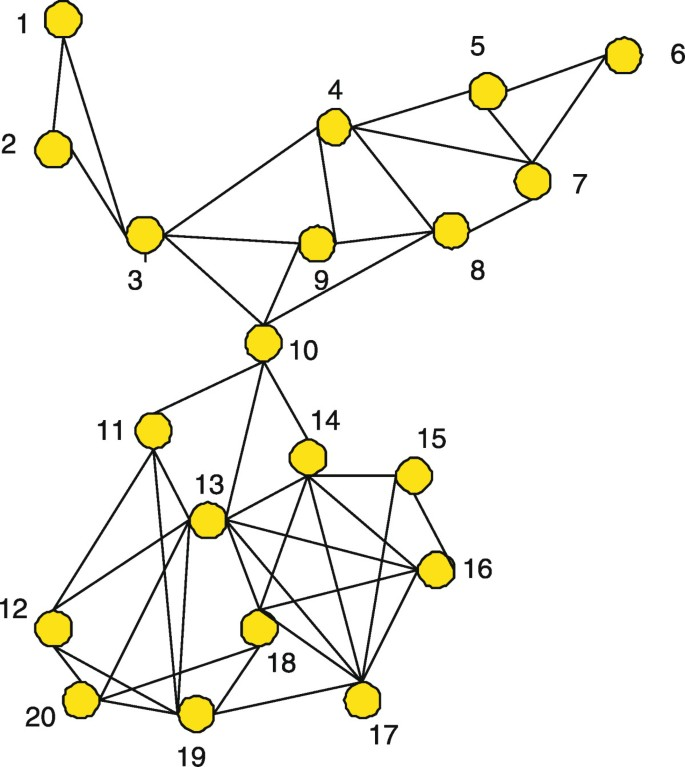Figure 2

### 6.1 Performance of the SVD and Cholesky algorithms

Initially, the two algorithms are used to identify an unknown parameter vector of length M = 4 in an environment with the two signal-to-noise ratios (SNR) of 10 and 20 dB. The two forgetting factors used are fixed at λ = {0.9,0.99}. The block size is taken as K = 8. The results for both algorithms are shown in Figures 3, 4, 5 and 6, for both diffusion (DRC, DRS) and no cooperation (NRC, NRS) cases. As can be seen from these figures, the Cholesky algorithm does not perform well with the smaller forgetting factor. However, the performance improves appreciably with an increase in the forgetting factor but its speed of convergence decreases significantly as well. However, the one main positive attribute of the Cholesky algorithm remains to be its low computational complexity. For the SVD algorithm, the performance improves slightly with an increase in forgetting factor but at a significant loss of convergence speed. These remarks have prompted us to further analyze the impact of the forgetting factor on the performance on these two algorithms, as discussed next.Figure 3Figure 4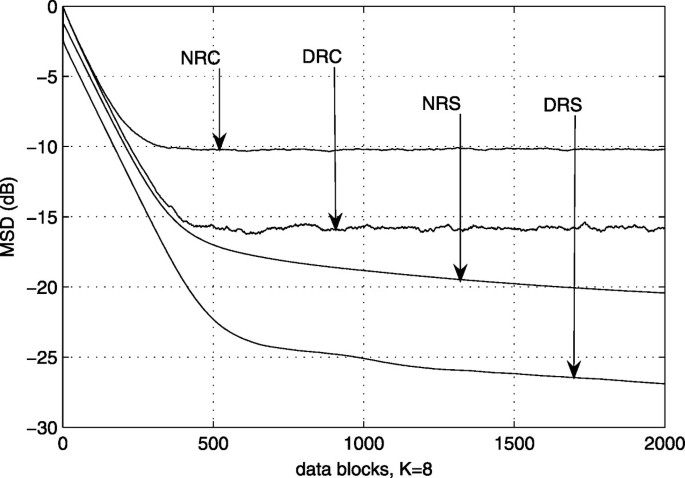Figure 5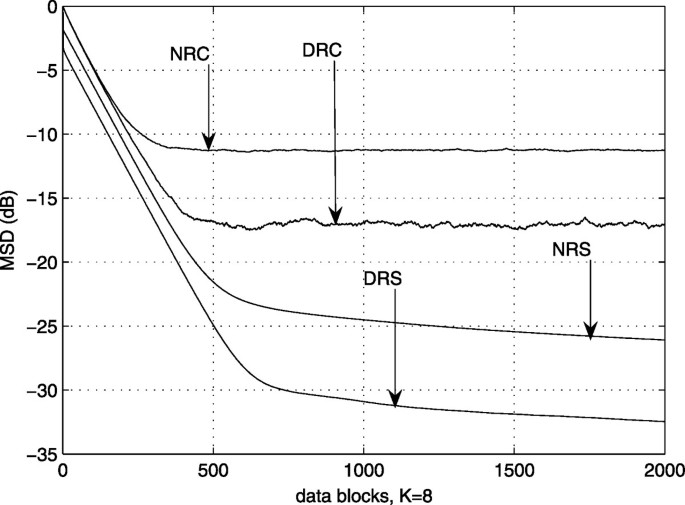Figure 6

### 6.2 Further simulation-based analysis of the effect of forgetting factor

Next, the performance of each algorithm is separately studied for different values of the forgetting factor. For the fixed forgetting factor case, the values taken are λ = {0.9,0.95,0.99} and the results are compared with those of the variable forgetting factor case. The SNR is chosen as 20 dB and the network size is taken to be 20 nodes. Figure 7 shows the results for the Cholesky factorization-based RC algorithm. It is seen that the performance improves as the forgetting factor is increased but the convergence slows down. The algorithm performs best when the forgetting factor is variable. The results for the SVD-based RS algorithm are shown in Figures 8, 9 and 10. Figure 8 shows the results for all three fixed forgetting factors as well as those for its variable one. However, as there is not much difference in performance between the four cases, Figure 8 is then zoomed in to see more clearly the algorithm’s transient and near-steady-state behavior. Figure 9 shows the result of this zooming effect. The speed of convergence is fastest for λ = 0.9 and slowest for λ = 0.99. For the variable forgetting factor (VFF) case, the speed is fast initially but then slows down with time. Figure 10 shows the behavior of the algorithm near steady-state. It is evident that the fixed case of λ = 0.99 would yield the lowest steady-state error whereas the VFF case would take the longest to reach the steady-state. Although the steady-state performance of the variable forgetting factor may be as good as for the case of λ=0.99 or even better, its speed of convergence is too slow.Figure 7Figure 8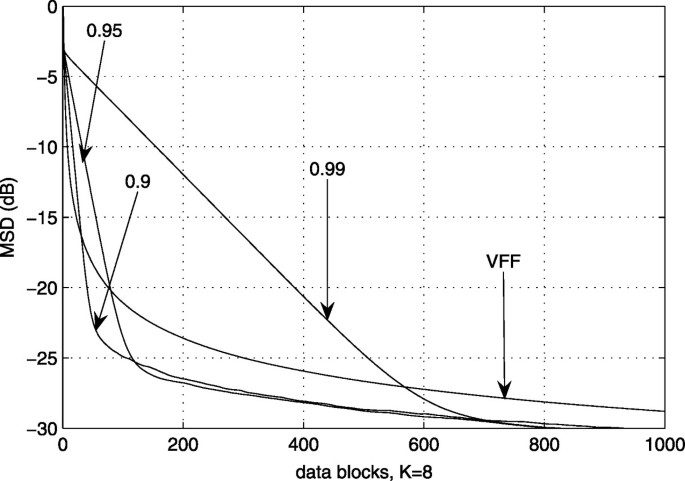Figure 9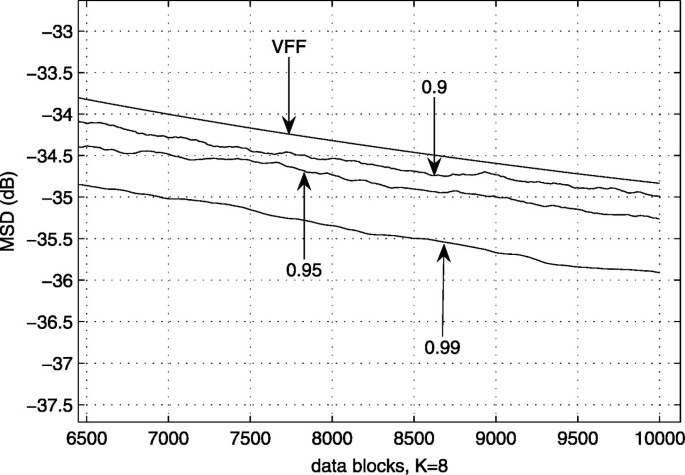Figure 10

### 6.3 Performance of the two algorithms using an optimal forgetting factor

From the results of Figures 7, 8, 9 and 10, it can easily be inferred that the Cholesky factorization-based approach yields the best results when the forgetting factor is varied whereas the SVD-based algorithm performs best if the forgetting factor is fixed. In order to have a fair performance comparison, the two algorithms need to be compared under conditions in which they both perform best. Figures 11 and 12 give the best performance results of the two algorithms, respectively. As can be seen from these two figures, at an SNR of 10 dB, the Cholesky-based DRC algorithm performs slightly better than the SVD-based RS algorithm without diffusion, whereas both SVD-based algorithms outperform the Cholesky-based algorithms at an SNR of 20 dB. However, the RC algorithm remains computationally less complex than the RS one. A final choice of either of these two algorithms will have to be based on a trade-off between their complexity and performance.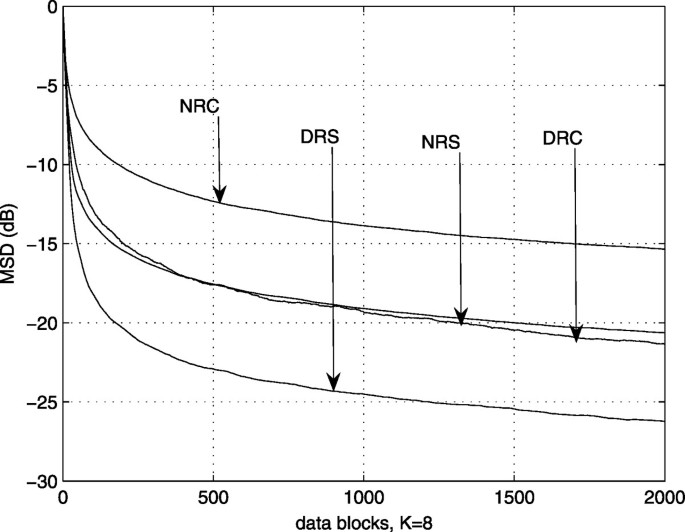Figure 11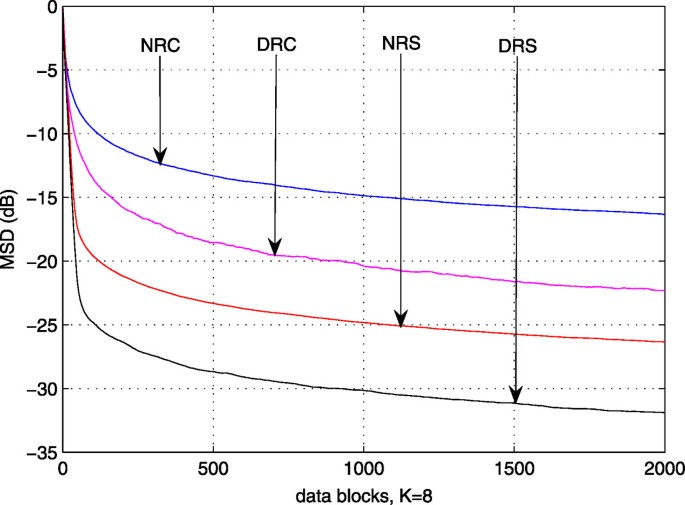Figure 12

It would be only fair to compare the performance of these algorithms with the original batch processing algorithms from  and . At an SNR of 10 dB, the MSE value for the SVD-based algorithm of  is −25.69 dB while that of the Cholesky-based algorithm from  is −17.79 dB. The corresponding numbers at an SNR of 20 are −31.38 and −18.36 dB, respectively. Comparing these results with the figures, we see that both recursive algorithms perform similar to their batch-processing counterparts. Furthermore, the diffusion algorithms perform better than the batch processing algorithms. The comparison results are tabulated in Table 3.

### 6.4 Effect of block size

Since it has been stated in  and  that the block size can affect the performance of the algorithm, the performance of our algorithms is also tested here for various block sizes. The block size is varied as K = {5,8,10,15,20} and the SNR is set to 20 dB. These settings are applied to both algorithms separately. Here it is important to note that as the size of the data block increases, the total amount of data required for the same number of blocks also increases. Figures 13 and 14 show the results for the RC algorithm and clearly demonstrate that the algorithm fails badly for K = 5. However, for the remaining block sizes, the algorithm’s performance remains almost unaffected by the block size changes. The convergence speeds are nearly the same (see Figure 13) and the performance at steady-state is similar as well for the remaining block sizes, with only a slight difference (see Figure 14). From Figure 14 it can be inferred that the best result, in every respect, is achieved when the block size is just large enough to achieve a full rank input data matrix (K=8 in this case), as expected. Thus, it is essential to estimate a tight upper bound for the size of the unknown vector in order to achieve good performance. Figures 15 and 16 show the results for the RS algorithm. Here, the performance improves gradually with an increase in block size. However, the speed of convergence is slow for a large block size (see Figure 15) even though a larger block size gives better performance at steady-state (see Figure 16). Again it can be inferred that it is best to keep the block size reasonably small in order to achieve a good trade off between performance and speed of convergence, especially when taking into account the fact that a larger block size would mean sensing more data for the same number of blocks.Figure 13Figure 14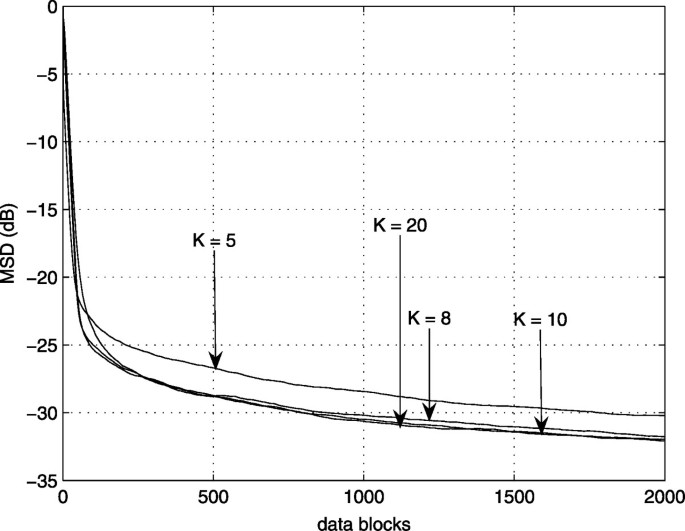Figure 15Figure 16

### 6.5 Effect of network size

Here the effect of the size of the network on the performance of the algorithms is discussed. For this purpose, the size of the network is varied over the range N = {10−50} while the forgetting factor is kept fixed at λ = 0.9 for the RS algorithm and made variable for the RC algorithm. The block size is taken as K = 8. This performance comparison is also carried out for both algorithms separately. The number of neighbors for each node is increased gradually as the size of the network is increased. Figures 17 and 18 show results for the RC algorithm. The performance is poor for N = 10 but improves as N increases. The initial speed of convergence is similar for various network sizes as can be seen in Figure 17 but, near steady-state, the networks with large sizes show a slight improvement in performance, as shown in Figure 18. Figures 19 and 20 show the results for the RS algorithm. Here the trend is slightly different. It can be seen that the initial speed of convergence improves with an increase in N (see Figure 19) but the improvement in performance is slightly smaller near steady-state (see Figure 20). Also, the difference in performance is smaller for larger networks, which is as expected.Figure 17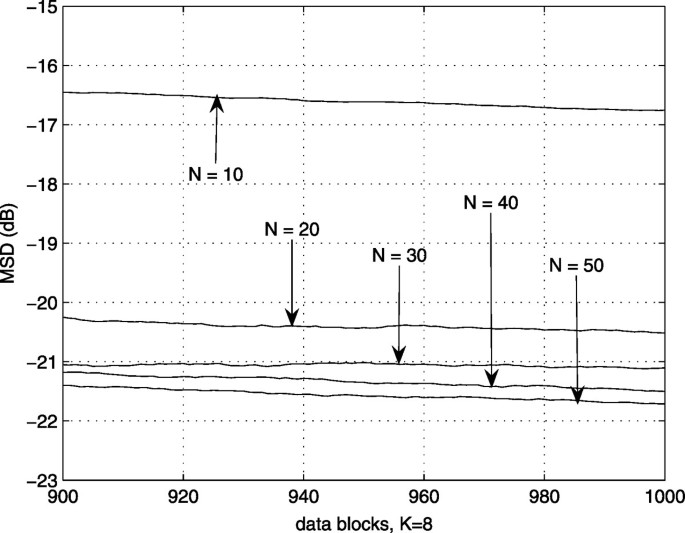Figure 18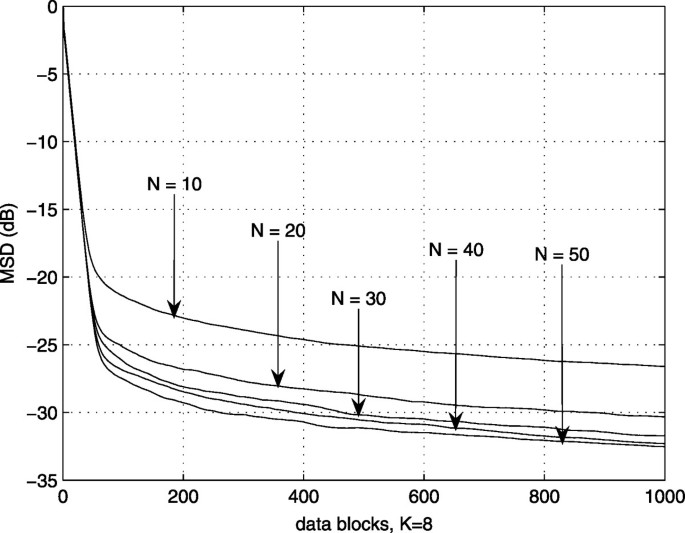Figure 19Figure 20

### 6.6 Effect of node malfunction

Finally, it is shown how the performance can be affected if one or more nodes malfunction. Two different network sizes are chosen in two different SNR scenarios to show how performance gets affected by node malfunction or failure. First, a network of 20 nodes is used and five nodes are switched off. Here, switching off a node means that it stops to participate in any further estimation process. The functioning nodes then re-calibrate the weights for the remaining neighbors while the weights for the failed nodes are set to zero. The nodes with the maximum number of neighbors are switched off to see how seriously the network performance might be affected. Results are shown for both SNRs, 10 and 20 dB, in Figures 21 and 22 respectively. The network size is then increased to 50 nodes with about a quarter of the nodes (13) switched off. The corresponding results are shown in Figures 23 and 24. The RC algorithm’s performance is worst affected at SNR = 10 dB but remains almost unaffected at SNR = 20 dB, with the small difference in performance getting even smaller when the network size is increased. Under similar test conditions as for the RC algorithm, the degradation of the SVD-based algorithm’s performance was found to be similar to that of the RC’s in all test cases. This clearly shows that the SVD-based algorithm is also strongly dependent on the connectivity of the nodes. As expected, the overall performance improves with an increase in network size. The effect of switched-off nodes on the performance of both algorithms, however, is similar when the ratio of switched-off nodes with maximum numbers of neighbor nodes to the total number nodes is the same.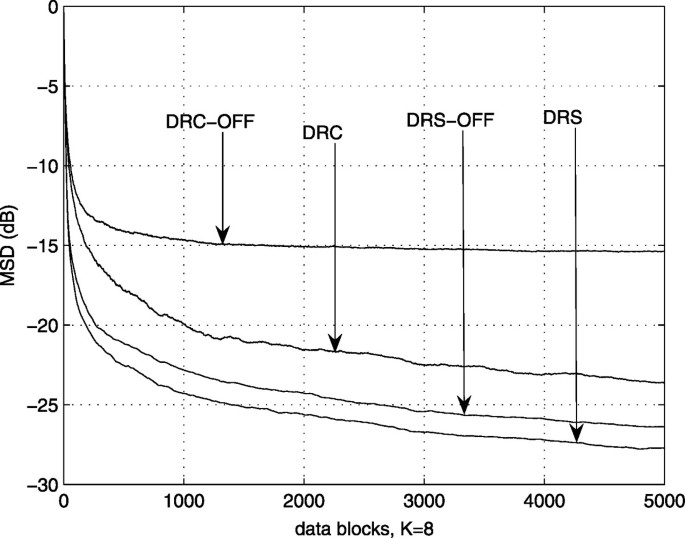Figure 21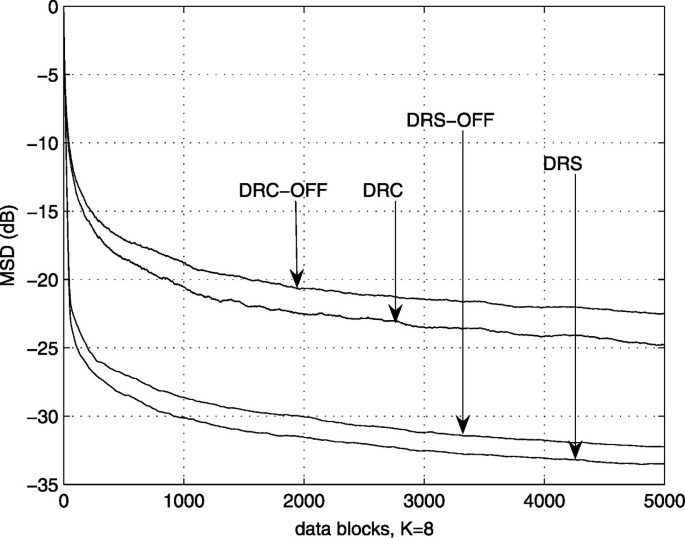Figure 22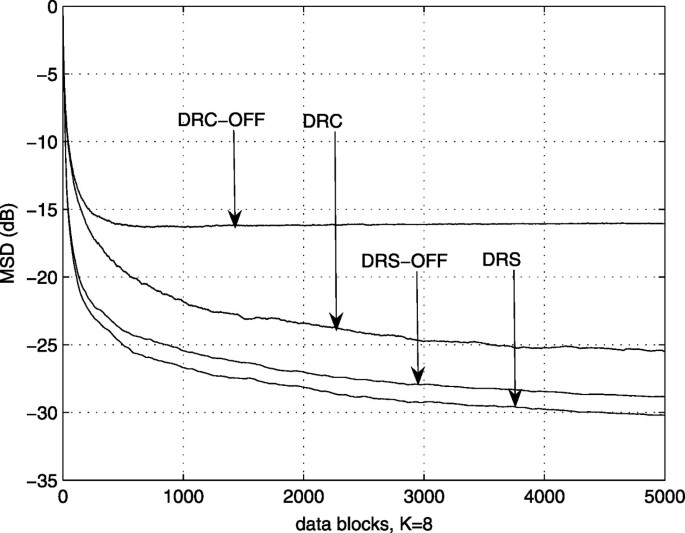Figure 23Figure 24

## 7 Conclusion

This work develops blind block recursive least squares algorithms based on Cholesky factorization and singular value decomposition (SVD). The algorithms are then used to estimate an unknown vector of interest in a wireless sensor network using cooperation between neighboring sensor nodes. Incorporating the algorithms in the sensor networks creates new diffusion-based algorithms, which are shown to perform much better than their non-diffusion-based counterparts. The new algorithms have been tested using both a variable as well as a fixed forgetting factor. The two developed algorithms are named diffusion blind block recursive Cholesky (DRC) and diffusion blind block recursive SVD (DRS) algorithms. Extensive simulation work comparing the two algorithms under different scenarios revealed that the DRS algorithm performs much better than the DRC algorithm but at the cost of a higher computational complexity. Also, of the two algorithms, the DRC algorithm performs better when the forgetting factor is variable whereas the DRS algorithm gives better results with a fixed forgetting factor. In the case of DRS, the value of the forgetting factor does not effect the overall performance a great deal except for a slight variation in convergence speed and steady-state performance. It was also seen that the size of the data block has an effect on the performance of the two algorithms. The speed of convergence slows down with an increasing block size which means an increasing amount of data to be processed. A block size increase, however, does not necessarily improve performance. It was found that, in general, a small block size gives a better performance. Therefore, it is essential to estimate a very low upper bound to the size of the unknown vector so that the data block size to be used is not unnecessarily large. Next, it was noticed that an increase in the network size improves performance but the improvement gradually decreases with an increasing network size. Moreover, it was shown that switching off some nodes with the largest neighborhoods can slightly degrade the performance of the algorithm. Finally at low SNRs, the Cholesky-based algorithm suffers from a severe degradation, whereas the SVD-based one only experiences a slight degradation.

## References

1. 1.

Sayed AH, Lopes CG: Distributed recursive least-squares strategies over adaptive networks. In Proceedings of the 40th Asilomar Conference on Signals, Systems, Computers. Monterey, CA; 2006:233-237.

2. 2.

Lopes CG, Sayed AH: Incremental adaptive strategies over distributed networks. IEEE Trans. Signal Process 2007, 55: 4064-4077.

3. 3.

Lopes CG, Sayed AH: Diffusion least-mean squares over adaptive networks: formulation and performance analysis. IEEE Trans. Signal Process 2008, 56(7):3122-3136.

4. 4.

Schizas ID, Mateos G, Giannakis GB: Distributed LMS for consensus-based in-network adaptive processing. IEEE Trans. Signal Process 2009, 57(6):2365-2382.

5. 5.

Bin Saeed MO, Zerguine A, Zummo SA: A variable step-size strategy for distributed estimation over distributed networks. Eur. J. Adv. Signal Process 2013, 2013: 135. 10.1186/1687-6180-2013-135

6. 6.

Sato Y: A method of self-recovering equalization for multilevel amplitude-modulation. IEEE Trans. Commun 1975, COM-23(6):679-682.

7. 7.

Proakis J: Digital Communications. McGraw-Hill, New York; 2000.

8. 8.

Tong L, Perreau S: Multichannel blind identification: from subspace to maximum likelihood methods. Proc. IEEE 1998, 86(10):1951-1968. 10.1109/5.720247

9. 9.

Xu G, Liu H, Tong L, Kailath T: A least-squares approach to blind channel identification. IEEE Trans. Signal Process 1995, 43(12):2982-2993. 10.1109/78.476442

10. 10.

Abed-Meraim K, Qiu W, Hua Y: Blind system identification. IEEE Trans. Signal Process 1997, 45(3):770-773. 10.1109/78.558501

11. 11.

Scaglione A, Giannakis GB, Barbarossa S: Redundant filterbank precoders and equalizers part II: blind channel estimation, synchronization, and direct equalization. IEEE Tran. Signal Proc 1999, 47(7):2007-2022. 10.1109/78.771048

12. 12.

Manton JH, Neumann WD: Totally blind channel identification by exploiting guard intervals. Syst. Control Lett 2003, 48(2):113-119. 10.1016/S0167-6911(02)00278-5

13. 13.

Pham DH, Manton JH: A subspace algorithm for guard interval based channel identification and source recovery requiring just two received blocks. In Proceedings of the IEEE ICASSP ’03. Hong Kong; 2003:317-320.

14. 14.

Su B, Vaidyanathan PP: A generalized algorithm for blind channel identification with linear redundant precoders. Eur. J. Adv. Signal Proc 2007, 2007: 1-13. Article ID 25672

15. 15.

Choi J, Lim C-C: A cholesky factorization based approach for blind FIR channel identification. IEEE Tran. Signal Proc 2008, 56(4):1730-1735.

16. 16.

Cattivelli F, Sayed AH: Diffusion LMS strategies for distributed estimation. IEEE Trans. Signal Process 2010, 58(3):1035-1048.

Download references

## Acknowledgments

The authors acknowledge the support provided by the Deanship of Scientific Research (DSR) at KFUPM under Research Grants RG1216, RG1112, SB101024, and FT100012.

## Author information

Authors

### Corresponding author

Correspondence to Azzedine Zerguine.

## Additional information

### Competing interests

The authors declare that they have no competing interests.

## Authors’ original submitted files for images

Below are the links to the authors’ original submitted files for images.

## Rights and permissions

Reprints and Permissions